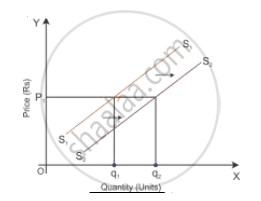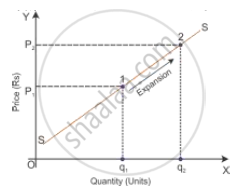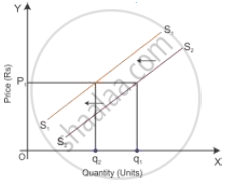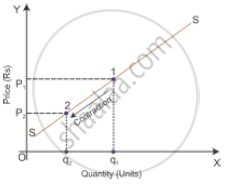# Explain the difference between “Shift of Supply Curve” and “Movement along Supply Curve”. State one factor responsible for each. Use diagrams. - Economics

Explain the difference between “Shift of Supply Curve” and “Movement along Supply Curve”. State one factor responsible for each. Use diagrams.

#### Solution

 Basis Shift in Supply Curve Movement Along Supply Curve Meaning Assuming that the price remains constant, there is change in the supply because of change in other variables such as technological change, input prices, unit tax and price of other related goods. Assuming that other variables remain constant, there is a change in quantity supplied because of the change in the own price of a good. Functional relation Q_x=f(barP_x,P_y,P_F,T,G,G_p,N_F) where barP_x="Own price of a good" Py = Price of related goods PF = Price of factors of production T = State of technology G = Goal of the firm Gp = Government policy NF = Number of firms Q_x=f(barP_x,barP_y,barT,bar(P_F),barG,barG_p,bar(N_F)) where barP_x="Own price of a good" barP_y="Price of related goods" bar(P_F)="Price of factors of production" barT="State of technology" barG="Goal of the firm" barG_p="Government policy" barN_"F"="Number of firms" Shift and Movement The supply curve of the firm will shift rightward or leftward. The supply curve of the firm will move upwards or downwards. Types Shift in the supply curve— increase in supply and decrease in supply. Movement along a supply curve—expansion of supply and contraction of supply. Diagram for increase/expansion Increase in supplyExpansion in supplyDiagram for decrease/contraction Decrease in supplyContraction in supplyConcept: Movements Along and Shifts in Supply Curve
Is there an error in this question or solution?
2015-2016 (March) Foreign Set 1
Share